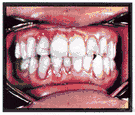calculus

(redirected from lung calculus)
Also found in: Thesaurus, Medical, Encyclopedia.

cal·cu·lus

(kăl′kyə-ləs)
n. pl. cal·cu·li (-lī′) or cal·cu·lus·es
1. Medicine An abnormal concretion in the body, usually formed of mineral salts and found in the gallbladder, kidney, or urinary bladder, for example.
2. Dentistry See tartar.
3. Mathematics
a. The branch of mathematics that deals with limits and the differentiation and integration of functions of one or more variables.
b. A method of analysis or calculation using a special symbolic notation.
c. The combined mathematics of differential calculus and integral calculus.
4. A system or method of calculation: "[a] dazzling grasp of the nation's byzantine budget calculus" (David M. Alpern).

[Latin, small stone used in reckoning; see calculate.]

calculus

(ˈkælkjʊləs)
n, pl -lusespl -li (-ˌlaɪ)
1. (Mathematics) a branch of mathematics, developed independently by Newton and Leibniz. Both differential calculus and integral calculus are concerned with the effect on a function of an infinitesimal change in the independent variable as it tends to zero
2. (Mathematics) any mathematical system of calculation involving the use of symbols
3. (Logic) logic an uninterpreted formal system. Compare formal language2
4. (Pathology) pathol a stonelike concretion of minerals and salts found in ducts or hollow organs of the body
[C17: from Latin: pebble, stone used in reckoning, from calx small stone, counter]

cal•cu•lus

(ˈkæl kyə ləs)

n., pl. -li (-ˌlaɪ)
-lus•es.
1. a method of calculation, esp. one of several highly systematic methods of treating problems by a special system of algebraic notations, as differential or integral calculus.
2. a stone, or concretion, formed in the gallbladder, kidney, or other part of the body.
3. a hard, yellowish to brownish black deposit on teeth formed largely through the calcification of dental plaque; tartar.
4. calculation: the calculus of political appeal.
[1610–20; < Latin: pebble, small stone (used in reckoning)]

cal·cu·lus

(kăl′kyə-ləs)
The branch of mathematics that finds the maximum or minimum values of functions by means of differentiation and integration. Calculus can be used to calculate such things as rates of change, the area bounded by curves, and the volume bounded by surfaces. See more at differentiation, integration.
Word History The branch of mathematics called calculus deals with problems that simple arithmetic or algebra cannot deal with, such as finding areas and volumes of unusual shapes and solids, and measuring rates of change. The word calculus comes from the Latin word that means "little stone, pebble." How did a word meaning "little stone" come to refer to this branch of mathematics? The answer comes from the counting practices of the ancient Romans over 2,000 years ago. They would add things up by using little pebbles or stones that represented particular numbers, as on an abacus. Later, the word calculus came to mean not just the pebble used in counting, but a counting system itself. Much more recently, it came to refer to the modern branch of mathematics, which was invented in the 17th century. The word calculus, incidentally, is also the source of the Latin word calculare, "to add up," which gives us our word calculate.

calculus

a branch of mathematics that treats the measurement of changing quantities, determining rates of change (differential calculus) and quantities under changing conditions (integral calculus).
ThesaurusAntonymsRelated WordsSynonymsLegend:
 Noun 1 calculus - a hard lump produced by the concretion of mineral salts; found in hollow organs or ducts of the body; "renal calculi can be very painful"concretionbladder stone, cystolith - a calculus formed in the bladderenterolith - a calculus occurring in the intestinesbilestone, gallstone - a calculus formed in the gall bladder or its ductskidney stone, nephrolith, renal calculus, urinary calculus - a calculus formed in the kidneyptyalith - calculus in a salivary glandrock, stone - a lump or mass of hard consolidated mineral matter; "he threw a rock at me"salivary calculus, sialolith - a stone formed in the salivary glandurolith - a urinary stone 2calculus - an incrustation that forms on the teeth and gumscrust, encrustation, incrustation - a hard outer layer that covers something 3 calculus - the branch of mathematics that is concerned with limits and with the differentiation and integration of functionsinfinitesimal calculusmath, mathematics, maths - a science (or group of related sciences) dealing with the logic of quantity and shape and arrangementpure mathematics - the branches of mathematics that study and develop the principles of mathematics for their own sake rather than for their immediate usefulnessanalysis - a branch of mathematics involving calculus and the theory of limits; sequences and series and integration and differentiationdifferential calculus, method of fluxions - the part of calculus that deals with the variation of a function with respect to changes in the independent variable (or variables) by means of the concepts of derivative and differentialintegral calculus - the part of calculus that deals with integration and its application in the solution of differential equations and in determining areas or volumes etc.calculus of variations - the calculus of maxima and minima of definite integrals
Translations
càlcul
počet
kalkulus
calcolotartarotartaro dentario

kamień nazębny
analys
kalkülüs

calculus

[ˈkælkjʊləs] N (calculuses or calculi (pl)) [ˈkælkjʊlaɪ] (Math) →
integral/differential calculuscálculo m integral/diferencial

calculus

[ˈkælkjʊləs] nanalyse f (mathématique), calcul m infinitésimal integral calculus, differential calculus

calculus

[ˈkælkjʊləs] ncalcolo
differential/integral calculus → calcolo differenziale/integrale

cal·cu·lus

n. cálculo, concreción o pequeña piedra que puede formarse en las secreciones y fluidos del organismo;
biliary ______ biliar;
calcium oxalate ______ de oxalato de calcio;
cystine ______ de cistina;
fibrin ______ de fibrina;
urinary ______ urinario.

calculus

n (stone) cálculo, piedra; (dent) cálculo, sarro (dental); renal — cálculo renal, piedra en el riñón
Site: Follow: Share:
Open / Close